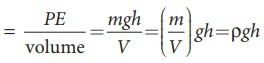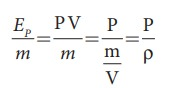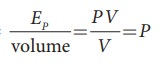Home | | Physics 11th std | Pressure, kinetic and potential energy of liquids

# Pressure, kinetic and potential energy of liquids

A liquid in a steady flow can possess three kinds of energy. They are (1) Kinetic energy, (2) Potential energy, and (3) Pressure energy, respectively.

Pressure, kinetic and potential energy of liquids

A liquid in a steady flow can possess three kinds of energy. They are (1) Kinetic energy, (2) Potential energy, and (3) Pressure energy, respectively.

i) Kinetic energy:â€‚ The kinetic energy of a liquid of mass m moving with a velocity is given byThe kinetic energy per unit mass =Similarly, the kinetic energy per unit volumeii) Potential energy: The potential energy of a liquid of mass m at a height h above the ground level is given by

PE = mgh

The potential energy per unit massSimilarly, the potential energy per unit volume =ii) Pressure energy: The energy acquired by a fluid by applying pressure on the fluid. We know that

Pressure = Force/Area â‡’ Force = Pressure Ă— Area

F Ă— d = (P A) Ă— d = P (A Ă— d)

F Ă— d = W = P V = pressure energy

Therefore, pressure energy, EP = PV The pressure energy per unit mass =Similarly,  the  potential  energy  per  unit volume =Study Material, Lecturing Notes, Assignment, Reference, Wiki description explanation, brief detail
11th Physics : UNIT 7 : Properties of Matter : Pressure, kinetic and potential energy of liquids |